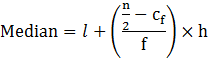Guru

# The following table gives the distribution of a life time of 400 neon lamps. Q.5

• 0

Give me the best and the simple way for solving the question from Statistics chapter of exercise 14.3 of question no,5. How i solve this problem in easy and simple way The following table gives the distribution of a life time of 400 neon lamps.

Share

Data:

n = 400 &n/2 = 200

Median class = 3000 – 3500

Therefore, l = 3000, Cf = 130,

f = 86 & h = 500Median = 3000 + ((200-130)/86) × 500

= 3000 + (35000/86)

= 3000 + 406.97

= 3406.97

Therefore, the median life time of the lamps = 3406.97 hours

• 0